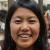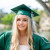## Document preview:

If a number does not have a decimal point, the zeros that are on the right side will determine if that number is significant or not. If a number has a decimal point, all the zeros that come to the right are considered as significant numbers. A significant number when recorded in a measurement will be equal to the number of digits that are known to have degrees of confidence. The last digit is usually an estimate or approximate. If the sensitivity of an equipment used in taking measurements is improved the precision of the significant figures will be increased. However, those that set the decimal point after a calculation are not deemed to be important. Trailing zeros that would not be needed in holding a decimal point are significant figures.If there is no decimal point in a measured value, all the number would be significant. Leading zeros in this case are not however significant. There are figures that represent a finite number of things for example the number of people in a room. Sp...

\$25
Thesis (40 pages)
Chemistry
Enter valid email

Significant FiguresLitTeacher1
4.5 (166 reviews)

4 in Chemistry

241 completed projects

## This might be interestingYoung H
5kevindeals
0